# SparkML之推荐算法（一）ALS

ALS(alternating least squares ):交替最小二乘法

---------------------------------------------------------------------

Matlab 主成分分析应用als

Spark源码

SparkML实验

---------------------------------------------------------------------

ALS在推荐系统中应用参考论文：见文献1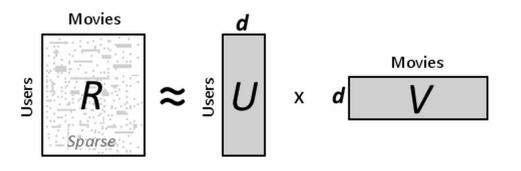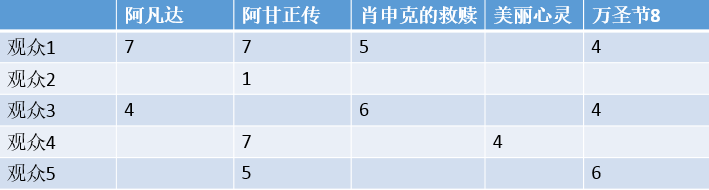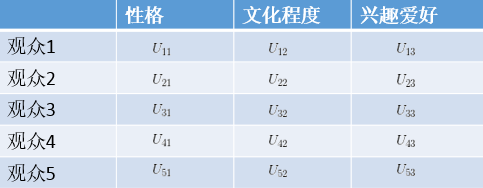V的矩阵如下：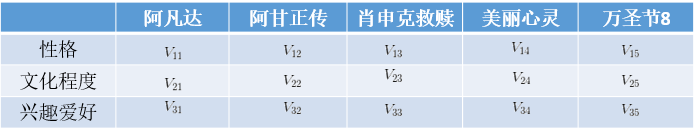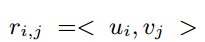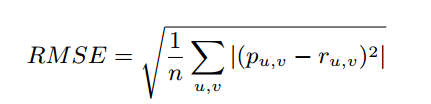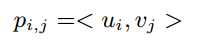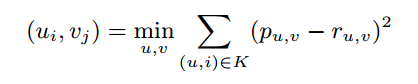（1）、我们所知道的K矩阵是稀疏矩阵（K就是R在U对V没有全部评价的矩阵）
（2）、K的大小远小于远小于R的密集对应的大小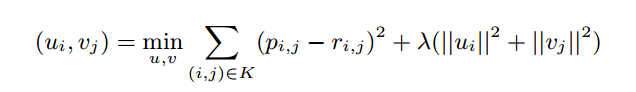note：其实后面的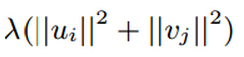是为了解决过拟合问题而增加的。

%目的
% 通过主成分分析，用ALS来优化，同时来得到潜在的评分，数据就是上面观众看电影数据
load Data.txt
R = Data;
[coeff1,score1,latent,tsquared,explained,mu1] = pca(R,'algorithm','als');
%% 参数
%coeff1  主成分系数
% 0.2851   -0.5043    0.8124   -0.0266
%  0.9230   -0.0764   -0.3655    0.0830
%  -0.1822   -0.4338   -0.1826    0.8602
%  -0.0890   -0.2844   -0.0782   -0.0986
%   0.1602    0.6861    0.4085    0.4927
%score1  主成分得分
% 3.1434   -2.0913   -0.1917   -0.0505
%  -3.1122    0.5615   -0.1839   -0.2997
%  -4.9612   -0.4934   -0.0388    0.2334
%  3.3091    1.5365   -0.4941    0.1154
%   1.6210    0.4868    0.9086    0.0014
%latent  主成分方差
%14.4394
%  1.8826
%   0.2854
%   0.0400
%tsquared Hotelling的T平方统计，在X每个观测
%3.2000
%   3.2000
%   3.2000
%   3.2000
%   3.2000
%explained 向量包含每个主成分解释的总方差的百分比
% 86.7366
%  11.3084
%  1.7145
%  0.2405
%mu1 返回的平均值
% 5.2035    3.8730    4.6740    4.7043    5.0344

%% 重建矩阵（预测）
p = score1*coeff1' + repmat(mu1,5,1)

%7.0000    7.0000    5.0000    5.0393    4.0000
%3.8915    1.0000    4.7733    4.8655    4.6982
%4.0000   -0.6348    6.0000    5.2662    4.0000
% 4.9677    7.0000    3.5939    4.0000    6.4738
%6.1583    5.0000    4.0027    4.3503    6.0000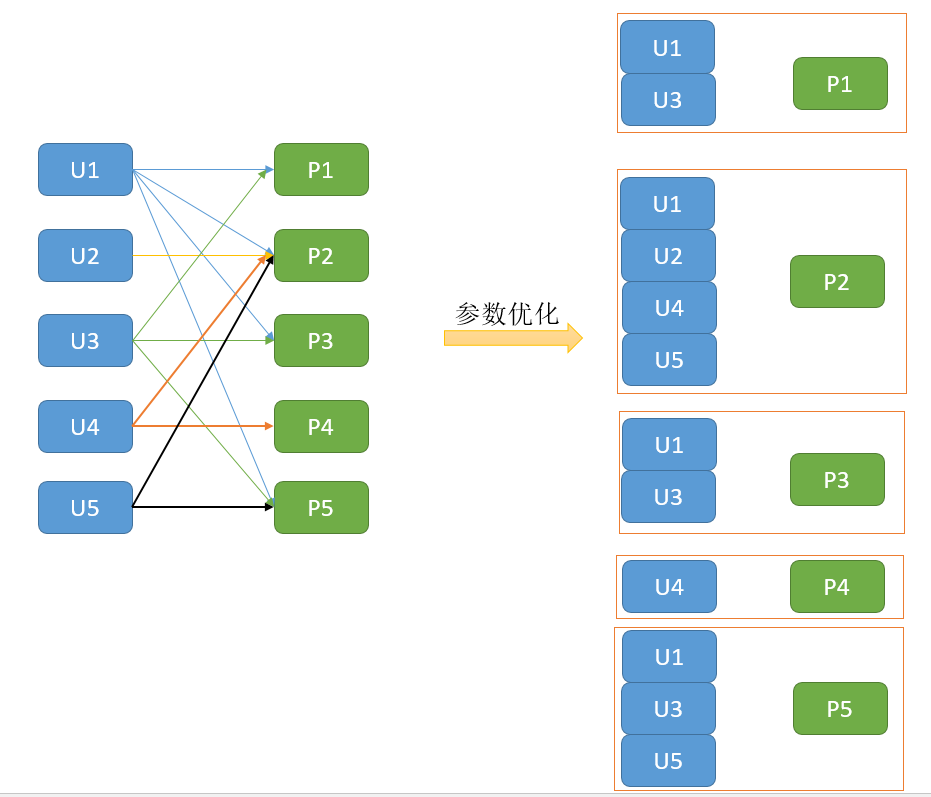/**
* 一个比Tuple3[Int, Int, Double]更加紧凑的class 用于表示评分
*/
@Since("0.8.0")
case class Rating @Since("0.8.0") (
@Since("0.8.0") user: Int,
@Since("0.8.0") product: Int,
@Since("0.8.0") rating: Double)

/**
* 交替最小二乘法的矩阵分解。
*
* ALS attempts to estimate the ratings matrix R as the product of two lower-rank matrices,
* X and Y, i.e. X * Yt = R. Typically these approximations are called 'factor' matrices.
* The general approach is iterative. During each iteration, one of the factor matrices is held
* constant, while the other is solved for using least squares. The newly-solved factor matrix is
* then held constant while solving for the other factor matrix.
*
* This is a blocked implementation of the ALS factorization algorithm that groups the two sets
* of factors (referred to as "users" and "products") into blocks and reduces communication by only
* sending one copy of each user vector to each product block on each iteration, and only for the
* product blocks that need that user's feature vector. This is achieved by precomputing some
* information about the ratings matrix to determine the "out-links" of each user (which blocks of
* products it will contribute to) and "in-link" information for each product (which of the feature
* vectors it receives from each user block it will depend on). This allows us to send only an
* array of feature vectors between each user block and product block, and have the product block
* find the users' ratings and update the products based on these messages.
*
* For implicit preference data, the algorithm used is based on
* "Collaborative Filtering for Implicit Feedback Datasets", available at
* [[http://dx.doi.org/10.1109/ICDM.2008.22]], adapted for the blocked approach used here.
*
* Essentially instead of finding the low-rank approximations to the rating matrix R,
* this finds the approximations for a preference matrix P where the elements of P are 1 if
* r > 0 and 0 if r <= 0. The ratings then act as 'confidence' values related to strength of
* indicated user
* preferences rather than explicit ratings given to items.
*/
@Since("0.8.0")
class ALS private (
private var numUserBlocks: Int,
private var numProductBlocks: Int,
private var rank: Int,
private var iterations: Int,
private var lambda: Double,
private var implicitPrefs: Boolean,
private var alpha: Double,
private var seed: Long = System.nanoTime()
) extends Serializable with Logging {

/**
* 构造一个默认参数的ALS的实例: {numBlocks: -1, rank: 10, iterations: 10,
* lambda: 0.01, implicitPrefs: false, alpha: 1.0}.
*/
@Since("0.8.0")
def this() = this(-1, -1, 10, 10, 0.01, false, 1.0)

/** 如果是true，做交替的非负最小二乘。. */
private var nonnegative = false

/** storage level for user/product in/out links */
private var intermediateRDDStorageLevel: StorageLevel = StorageLevel.MEMORY_AND_DISK
private var finalRDDStorageLevel: StorageLevel = StorageLevel.MEMORY_AND_DISK

/** checkpoint interval */
private var checkpointInterval: Int = 10

/**
* 设置用户模块和产品模块并行计算块的数量（假设设置为2，那么用户和产品的模块都是2个）,numBlocks=-1的时候表示自动配置模块数，默认情况下是 numBlocks=-1
*/
@Since("0.8.0")
def setBlocks(numBlocks: Int): this.type = {
require(numBlocks == -1 || numBlocks > 0,
s"Number of blocks must be -1 or positive but got ${numBlocks}") this.numUserBlocks = numBlocks this.numProductBlocks = numBlocks this } /** * 设置并行计算的用户的块的数量。 */ @Since("1.1.0") def setUserBlocks(numUserBlocks: Int): this.type = { require(numUserBlocks == -1 || numUserBlocks > 0, s"Number of blocks must be -1 or positive but got${numUserBlocks}")
this.numUserBlocks = numUserBlocks
this
}

/**
* 设置并行计算的产品的块的数量。
*/
@Since("1.1.0")
def setProductBlocks(numProductBlocks: Int): this.type = {
require(numProductBlocks == -1 || numProductBlocks > 0,
s"Number of product blocks must be -1 or positive but got ${numProductBlocks}") this.numProductBlocks = numProductBlocks this } /** 计算特征矩阵的秩（特征数），默认情况下为10 */ @Since("0.8.0") def setRank(rank: Int): this.type = { require(rank > 0, s"Rank of the feature matrices must be positive but got${rank}")
this.rank = rank
this
}

/** 设置要运行的迭代次数。默人为10次 */
@Since("0.8.0")
def setIterations(iterations: Int): this.type = {
require(iterations >= 0,
s"Number of iterations must be nonnegative but got ${iterations}") this.iterations = iterations this } /** 集的正则化参数，λ. 默认为 0.01. */ @Since("0.8.0") def setLambda(lambda: Double): this.type = { require(lambda >= 0.0, s"Regularization parameter must be nonnegative but got${lambda}")
this.lambda = lambda
this
}

/** 设置是否使用隐式偏好。Default: false. */
@Since("0.8.1")
def setImplicitPrefs(implicitPrefs: Boolean): this.type = {
this.implicitPrefs = implicitPrefs
this
}

/**
* Sets the constant used in computing confidence in implicit ALS. Default: 1.0.
*/
@Since("0.8.1")
def setAlpha(alpha: Double): this.type = {
this.alpha = alpha
this
}

/** Sets a random seed to have deterministic results. */
@Since("1.0.0")
def setSeed(seed: Long): this.type = {
this.seed = seed
this
}

/**
* *
* 设置每一次迭代中的最小二乘法，是否都要非负约束
* Set whether the least-squares problems solved at each iteration should have
* nonnegativity constraints.
*/
@Since("1.1.0")
def setNonnegative(b: Boolean): this.type = {
this.nonnegative = b
this
}

/**
* :: DeveloperApi ::* 对每一个RDD在中间的缓存级别的选择
* Sets storage level for intermediate RDDs (user/product in/out links). The default value is
* MEMORY_AND_DISK. Users can change it to a serialized storage, e.g., MEMORY_AND_DISK_SER and
* set spark.rdd.compress to true to reduce the space requirement, at the cost of speed.
*/
@DeveloperApi
@Since("1.1.0")
def setIntermediateRDDStorageLevel(storageLevel: StorageLevel): this.type = {
require(storageLevel != StorageLevel.NONE,
"ALS is not designed to run without persisting intermediate RDDs.")
this.intermediateRDDStorageLevel = storageLevel
this
}

/**
* :: DeveloperApi ::
* Sets storage level for final RDDs (user/product used in MatrixFactorizationModel). The default
* value is MEMORY_AND_DISK. Users can change it to a serialized storage, e.g.
* MEMORY_AND_DISK_SER and set spark.rdd.compress to true to reduce the space requirement,
* at the cost of speed.
*/
@DeveloperApi
@Since("1.3.0")
def setFinalRDDStorageLevel(storageLevel: StorageLevel): this.type = {
this.finalRDDStorageLevel = storageLevel
this
}

/**
* Set period (in iterations) between checkpoints (default = 10). Checkpointing helps with
* recovery (when nodes fail) and StackOverflow exceptions caused by long lineage. It also helps
* with eliminating temporary shuffle files on disk, which can be important when there are many
* ALS iterations. If the checkpoint directory is not set in [[org.apache.spark.SparkContext]],
* this setting is ignored.
*/
@DeveloperApi
@Since("1.4.0")
//设置每隔多久 checkpoint一下
def setCheckpointInterval(checkpointInterval: Int): this.type = {
this.checkpointInterval = checkpointInterval
this
}

/**
* Run ALS with the configured parameters on an input RDD of [[Rating]] objects.
* Returns a MatrixFactorizationModel with feature vectors for each user and product.
*/
@Since("0.8.0")
//查看一开始给的Rating,這个RDD的形式内部数据如下：
//case class Rating @Since("0.8.0") (
//                                   @Since("0.8.0") user: Int,
//                                  @Since("0.8.0") product: Int,
//                                  @Since("0.8.0") rating: Double)
def run(ratings: RDD[Rating]): MatrixFactorizationModel = {
val sc = ratings.context

//分块设置，默认下：在并行度和rating的partitions的二分之一中选一个最大的
//          设置参数下：为numUserBlocks
val numUserBlocks = if (this.numUserBlocks == -1) {
math.max(sc.defaultParallelism, ratings.partitions.length / 2)
} else {
this.numUserBlocks
}
//分块设置，默认下：在并行度和rating的partitions的二分之一中选一个最大的
//          设置参数下：为numProductBlocks
val numProductBlocks = if (this.numProductBlocks == -1) {
math.max(sc.defaultParallelism, ratings.partitions.length / 2)
} else {
this.numProductBlocks
}

val (floatUserFactors, floatProdFactors) = NewALS.train[Int](
ratings = ratings.map(r => NewALS.Rating(r.user, r.product, r.rating.toFloat)),
rank = rank,
numUserBlocks = numUserBlocks,
numItemBlocks = numProductBlocks,
maxIter = iterations,
regParam = lambda,
implicitPrefs = implicitPrefs,
alpha = alpha,
nonnegative = nonnegative,
intermediateRDDStorageLevel = intermediateRDDStorageLevel,
finalRDDStorageLevel = StorageLevel.NONE,
checkpointInterval = checkpointInterval,
seed = seed)

val userFactors = floatUserFactors
.mapValues(_.map(_.toDouble))
.setName("users")
.persist(finalRDDStorageLevel)
val prodFactors = floatProdFactors
.mapValues(_.map(_.toDouble))
.setName("products")
.persist(finalRDDStorageLevel)
if (finalRDDStorageLevel != StorageLevel.NONE) {
userFactors.count()
prodFactors.count()
}
new MatrixFactorizationModel(rank, userFactors, prodFactors)
}

/**
* Java-friendly version of [[ALS.run]].
*/
@Since("1.3.0")
def run(ratings: JavaRDD[Rating]): MatrixFactorizationModel = run(ratings.rdd)
}

/**
* Top-level methods for calling Alternating Least Squares (ALS) matrix factorization.
*/
@Since("0.8.0")
object ALS {
/**
* Train a matrix factorization model given an RDD of ratings by users for a subset of products.
* The ratings matrix is approximated as the product of two lower-rank matrices of a given rank
* (number of features). To solve for these features, ALS is run iteratively with a configurable
* level of parallelism.
*
* @param ratings    RDD of [[Rating]] objects with userID, productID, and rating
* @param rank       number of features to use
* @param iterations number of iterations of ALS
* @param lambda     regularization parameter
* @param blocks     level of parallelism to split computation into
* @param seed       random seed for initial matrix factorization model
*/
@Since("0.9.1")
def train(
ratings: RDD[Rating],
rank: Int,
iterations: Int,
lambda: Double,
blocks: Int,
seed: Long
): MatrixFactorizationModel = {
new ALS(blocks, blocks, rank, iterations, lambda, false, 1.0, seed).run(ratings)
}

/**
* Train a matrix factorization model given an RDD of ratings by users for a subset of products.
* The ratings matrix is approximated as the product of two lower-rank matrices of a given rank
* (number of features). To solve for these features, ALS is run iteratively with a configurable
* level of parallelism.
*
* @param ratings    RDD of [[Rating]] objects with userID, productID, and rating
* @param rank       number of features to use
* @param iterations number of iterations of ALS
* @param lambda     regularization parameter
* @param blocks     level of parallelism to split computation into
*/
@Since("0.8.0")
def train(
ratings: RDD[Rating],
rank: Int,
iterations: Int,
lambda: Double,
blocks: Int
): MatrixFactorizationModel = {
new ALS(blocks, blocks, rank, iterations, lambda, false, 1.0).run(ratings)
}

/**
* Train a matrix factorization model given an RDD of ratings by users for a subset of products.
* The ratings matrix is approximated as the product of two lower-rank matrices of a given rank
* (number of features). To solve for these features, ALS is run iteratively with a level of
* parallelism automatically based on the number of partitions in ratings.
*
* @param ratings    RDD of [[Rating]] objects with userID, productID, and rating
* @param rank       number of features to use
* @param iterations number of iterations of ALS
* @param lambda     regularization parameter
*/
@Since("0.8.0")
def train(ratings: RDD[Rating], rank: Int, iterations: Int, lambda: Double)
: MatrixFactorizationModel = {
train(ratings, rank, iterations, lambda, -1)
}

/**
* Train a matrix factorization model given an RDD of ratings by users for a subset of products.
* The ratings matrix is approximated as the product of two lower-rank matrices of a given rank
* (number of features). To solve for these features, ALS is run iteratively with a level of
* parallelism automatically based on the number of partitions in ratings.
*
* @param ratings    RDD of [[Rating]] objects with userID, productID, and rating
* @param rank       number of features to use
* @param iterations number of iterations of ALS
*/
@Since("0.8.0")
def train(ratings: RDD[Rating], rank: Int, iterations: Int)
: MatrixFactorizationModel = {
train(ratings, rank, iterations, 0.01, -1)
}

/**
* Train a matrix factorization model given an RDD of 'implicit preferences' given by users
* to some products, in the form of (userID, productID, preference) pairs. We approximate the
* ratings matrix as the product of two lower-rank matrices of a given rank (number of features).
* To solve for these features, we run a given number of iterations of ALS. This is done using
* a level of parallelism given by blocks.
*
* @param ratings    RDD of (userID, productID, rating) pairs
* @param rank       number of features to use
* @param iterations number of iterations of ALS
* @param lambda     regularization parameter
* @param blocks     level of parallelism to split computation into
* @param alpha      confidence parameter
* @param seed       random seed for initial matrix factorization model
*/
@Since("0.8.1")
def trainImplicit(
ratings: RDD[Rating],
rank: Int,
iterations: Int,
lambda: Double,
blocks: Int,
alpha: Double,
seed: Long
): MatrixFactorizationModel = {
new ALS(blocks, blocks, rank, iterations, lambda, true, alpha, seed).run(ratings)
}

/**
* Train a matrix factorization model given an RDD of 'implicit preferences' of users for a
* subset of products. The ratings matrix is approximated as the product of two lower-rank
* matrices of a given rank (number of features). To solve for these features, ALS is run
* iteratively with a configurable level of parallelism.
*
* @param ratings    RDD of [[Rating]] objects with userID, productID, and rating
* @param rank       number of features to use
* @param iterations number of iterations of ALS
* @param lambda     regularization parameter
* @param blocks     level of parallelism to split computation into
* @param alpha      confidence parameter
*/
@Since("0.8.1")
def trainImplicit(
ratings: RDD[Rating],
rank: Int,
iterations: Int,
lambda: Double,
blocks: Int,
alpha: Double
): MatrixFactorizationModel = {
new ALS(blocks, blocks, rank, iterations, lambda, true, alpha).run(ratings)
}

/**
* Train a matrix factorization model given an RDD of 'implicit preferences' of users for a
* subset of products. The ratings matrix is approximated as the product of two lower-rank
* matrices of a given rank (number of features). To solve for these features, ALS is run
* iteratively with a level of parallelism determined automatically based on the number of
* partitions in ratings.
*
* @param ratings    RDD of [[Rating]] objects with userID, productID, and rating
* @param rank       number of features to use
* @param iterations number of iterations of ALS
* @param lambda     regularization parameter
* @param alpha      confidence parameter
*/
@Since("0.8.1")
def trainImplicit(ratings: RDD[Rating], rank: Int, iterations: Int, lambda: Double, alpha: Double)
: MatrixFactorizationModel = {
trainImplicit(ratings, rank, iterations, lambda, -1, alpha)
}

/**
* Train a matrix factorization model given an RDD of 'implicit preferences' of users for a
* subset of products. The ratings matrix is approximated as the product of two lower-rank
* matrices of a given rank (number of features). To solve for these features, ALS is run
* iteratively with a level of parallelism determined automatically based on the number of
* partitions in ratings.
*
* @param ratings    RDD of [[Rating]] objects with userID, productID, and rating
* @param rank       number of features to use
* @param iterations number of iterations of ALS
*/
@Since("0.8.1")
def trainImplicit(ratings: RDD[Rating], rank: Int, iterations: Int)
: MatrixFactorizationModel = {
trainImplicit(ratings, rank, iterations, 0.01, -1, 1.0)
}
}


MatrixFactorizationModel类

/**
*矩阵分解模型
*
* Note:如果你直接用构造函数来创建模型，请注意，快速预测需要缓存user/product,
*
* @param rank 秩
* @param userFeatures RDD的元组，每个元组都有计算后的userId 和 它features
* @param productFeatures RDD的元组，每个元组都有计算后的productId 和 它features
*/
@Since("0.8.0")
class MatrixFactorizationModel @Since("0.8.0") (
@Since("0.8.0") val rank: Int,
@Since("0.8.0") val userFeatures: RDD[(Int, Array[Double])],
@Since("0.8.0") val productFeatures: RDD[(Int, Array[Double])])
extends Saveable with Serializable with Logging {

require(rank > 0)
validateFeatures("User", userFeatures)
validateFeatures("Product", productFeatures)

/**验证因素，并提醒用户，如果有性能问题。 */
private def validateFeatures(name: String, features: RDD[(Int, Array[Double])]): Unit = {
require(features.first()._2.length == rank,
s"$name feature dimension does not match the rank$rank.")
if (features.partitioner.isEmpty) {
logWarning(s"$name factor does not have a partitioner. " + "Prediction on individual records could be slow.") } if (features.getStorageLevel == StorageLevel.NONE) { logWarning(s"$name factor is not cached. Prediction could be slow.")
}
}

/** 预测一个用户对一个产品的评价. */
@Since("0.8.0")
def predict(user: Int, product: Int): Double = {
val userVector = userFeatures.lookup(user).head
val productVector = productFeatures.lookup(product).head
blas.ddot(rank, userVector, 1, productVector, 1)
}

/**
* 输入usersProducts，返回用户和产品的近似数，這个方法是基于countApproxDistinct
*
* @param usersProducts  RDD of (user, product) pairs.
* @return 用户和产品的近似值。
*/
private[this] def countApproxDistinctUserProduct(usersProducts: RDD[(Int, Int)]): (Long, Long) = {
val zeroCounterUser = new HyperLogLogPlus(4, 0)
val zeroCounterProduct = new HyperLogLogPlus(4, 0)
val aggregated = usersProducts.aggregate((zeroCounterUser, zeroCounterProduct))(
(hllTuple: (HyperLogLogPlus, HyperLogLogPlus), v: (Int, Int)) => {
hllTuple._1.offer(v._1)
hllTuple._2.offer(v._2)
hllTuple
},
(h1: (HyperLogLogPlus, HyperLogLogPlus), h2: (HyperLogLogPlus, HyperLogLogPlus)) => {
h1._1.addAll(h2._1)
h1._2.addAll(h2._2)
h1
})
(aggregated._1.cardinality(), aggregated._2.cardinality())
}

/**
* 预测多个用户对产品的评价。
*输出的RDD和输入的RDD元素一一对应 （包括所有副本）除非用户或产品中缺少训练集。
* @param usersProducts  RDD of (user, product) pairs.
* @return RDD of Ratings.
*/
@Since("0.9.0")
def predict(usersProducts: RDD[(Int, Int)]): RDD[Rating] = {
// Previously the partitions of ratings are only based on the given products.
// So if the usersProducts given for prediction contains only few products or
// even one product, the generated ratings will be pushed into few or single partition
// and can't use high parallelism.
// Here we calculate approximate numbers of users and products. Then we decide the
// partitions should be based on users or products.
val (usersCount, productsCount) = countApproxDistinctUserProduct(usersProducts)

if (usersCount < productsCount) {
val users = userFeatures.join(usersProducts).map {
case (user, (uFeatures, product)) => (product, (user, uFeatures))
}
users.join(productFeatures).map {
case (product, ((user, uFeatures), pFeatures)) =>
Rating(user, product, blas.ddot(uFeatures.length, uFeatures, 1, pFeatures, 1))
}
} else {
val products = productFeatures.join(usersProducts.map(_.swap)).map {
case (product, (pFeatures, user)) => (user, (product, pFeatures))
}
products.join(userFeatures).map {
case (user, ((product, pFeatures), uFeatures)) =>
Rating(user, product, blas.ddot(uFeatures.length, uFeatures, 1, pFeatures, 1))
}
}
}

/**
* Java-friendly version of [[MatrixFactorizationModel.predict]].
*/
@Since("1.2.0")
def predict(usersProducts: JavaPairRDD[JavaInteger, JavaInteger]): JavaRDD[Rating] = {
predict(usersProducts.rdd.asInstanceOf[RDD[(Int, Int)]]).toJavaRDD()
}

/**
* 向用户推荐产品。
*
* @param user the user to recommend products to
* @param num how many products to return. The number returned may be less than this.
* @return [[Rating]] objects, each of which contains the given user ID, a product ID, and a
*  "score" in the rating field. Each represents one recommended product, and they are sorted
*  by score, decreasing. The first returned is the one predicted to be most strongly
*  recommended to the user. The score is an opaque value that indicates how strongly
*  recommended the product is.
*/
@Since("1.1.0")
def recommendProducts(user: Int, num: Int): Array[Rating] =
MatrixFactorizationModel.recommend(userFeatures.lookup(user).head, productFeatures, num)
.map(t => Rating(user, t._1, t._2))

/**
* 推荐用户给产品. 也就是说，看看那些用户对這个产品感兴趣
*
* @param product 产品推荐用户
* @param num 设定返回多少个用户，实际返回的大小有可能少于设定的值
* @return [[Rating]] objects, 其中每一个包含用户的ID、产品ID，和一个得分，每个表示一个推荐的用户，并且它们按从大到小的分数排序，第一次返回的是一个预测是最建议的产品
*/
@Since("1.1.0")
def recommendUsers(product: Int, num: Int): Array[Rating] =
MatrixFactorizationModel.recommend(productFeatures.lookup(product).head, userFeatures, num)
.map(t => Rating(t._1, product, t._2))

protected override val formatVersion: String = "1.0"

/**
* 输入路径、保持模型
*
* This saves:
*  - human-readable (JSON) model metadata to path/metadata/
*  - Parquet formatted data to path/data/
*
* The model may be loaded using [[Loader.load]].
*
* @param sc  Spark context used to save model data.
* @param path  Path specifying the directory in which to save this model.
*              If the directory already exists, this method throws an exception.
*/
@Since("1.3.0")
override def save(sc: SparkContext, path: String): Unit = {
MatrixFactorizationModel.SaveLoadV1_0.save(this, path)
}

/**
* 为所有用户推荐top products。
*
* @param num 为每个用户返回多少产品
* @return [(Int, Array[Rating])] objects, where every tuple contains a userID and an array of
* rating objects which contains the same userId, recommended productID and a "score" in the
* rating field. Semantics of score is same as recommendProducts API
*
*/
@Since("1.4.0")
def recommendProductsForUsers(num: Int): RDD[(Int, Array[Rating])] = {
MatrixFactorizationModel.recommendForAll(rank, userFeatures, productFeatures, num).map {
case (user, top) =>
val ratings = top.map { case (product, rating) => Rating(user, product, rating) }
(user, ratings)
}
}

/**
* 为所有产品推荐 top users
*
* @param num how many users to return for every product.
* @return [(Int, Array[Rating])] objects, where every tuple contains a productID and an array
* of rating objects which contains the recommended userId, same productID and a "score" in the
* rating field. Semantics of score is same as recommendUsers API
*/
@Since("1.4.0")
def recommendUsersForProducts(num: Int): RDD[(Int, Array[Rating])] = {
MatrixFactorizationModel.recommendForAll(rank, productFeatures, userFeatures, num).map {
case (product, top) =>
val ratings = top.map { case (user, rating) => Rating(user, product, rating) }
(product, ratings)
}
}
}

@Since("1.3.0")
object MatrixFactorizationModel extends Loader[MatrixFactorizationModel] {

import org.apache.spark.mllib.util.Loader._

/**
* 对单个用户（或产品）进行推荐
*/
private def recommend(
recommendToFeatures: Array[Double],
recommendableFeatures: RDD[(Int, Array[Double])],
num: Int): Array[(Int, Double)] = {
val scored = recommendableFeatures.map { case (id, features) =>
(id, blas.ddot(features.length, recommendToFeatures, 1, features, 1))
}
scored.top(num)(Ordering.by(_._2))
}

/**
* 对所有用户（或产品）进行推荐
* @param rank rank
* @param srcFeatures src features to receive recommendations
* @param dstFeatures dst features used to make recommendations
* @param num number of recommendations for each record
* @return an RDD of (srcId: Int, recommendations), where recommendations are stored as an array
*         of (dstId, rating) pairs.
*/
private def recommendForAll(
rank: Int,
srcFeatures: RDD[(Int, Array[Double])],
dstFeatures: RDD[(Int, Array[Double])],
num: Int): RDD[(Int, Array[(Int, Double)])] = {
val srcBlocks = blockify(rank, srcFeatures)
val dstBlocks = blockify(rank, dstFeatures)
val ratings = srcBlocks.cartesian(dstBlocks).flatMap {
case ((srcIds, srcFactors), (dstIds, dstFactors)) =>
val m = srcIds.length
val n = dstIds.length
val ratings = srcFactors.transpose.multiply(dstFactors)
val output = new Array[(Int, (Int, Double))](m * n)
var k = 0
ratings.foreachActive { (i, j, r) =>
output(k) = (srcIds(i), (dstIds(j), r))
k += 1
}
output.toSeq
}
ratings.topByKey(num)(Ordering.by(_._2))
}

/**
* Blockifies features to use Level-3 BLAS.
*/
private def blockify(
rank: Int,
features: RDD[(Int, Array[Double])]): RDD[(Array[Int], DenseMatrix)] = {
val blockSize = 4096 // TODO: tune the block size
val blockStorage = rank * blockSize
features.mapPartitions { iter =>
iter.grouped(blockSize).map { grouped =>
val ids = mutable.ArrayBuilder.make[Int]
ids.sizeHint(blockSize)
val factors = mutable.ArrayBuilder.make[Double]
factors.sizeHint(blockStorage)
var i = 0
grouped.foreach { case (id, factor) =>
ids += id
factors ++= factor
i += 1
}
(ids.result(), new DenseMatrix(rank, i, factors.result()))
}
}
}

/**
* 输入模型的路径，加载這个模型
*
* The model should have been saved by [[Saveable.save]].
*
* @param sc  Spark context used for loading model files.
* @param path  Path specifying the directory to which the model was saved.
* @return  Model instance
*/
@Since("1.3.0")
override def load(sc: SparkContext, path: String): MatrixFactorizationModel = {
val (loadedClassName, formatVersion, _) = loadMetadata(sc, path)
val classNameV1_0 = SaveLoadV1_0.thisClassName
(loadedClassName, formatVersion) match {
case (className, "1.0") if className == classNameV1_0 =>
SaveLoadV1_0.load(sc, path)
case _ =>
throw new IOException("MatrixFactorizationModel.load did not recognize model with" +
s"(class: $loadedClassName, version:$formatVersion). Supported:\n" +
s"  (\$classNameV1_0, 1.0)")
}
}

private[recommendation]
object SaveLoadV1_0 {

private val thisFormatVersion = "1.0"

private[recommendation]
val thisClassName = "org.apache.spark.mllib.recommendation.MatrixFactorizationModel"

/**
* Saves a [[MatrixFactorizationModel]], where user features are saved under data/users and
* product features are saved under data/products.
*/
def save(model: MatrixFactorizationModel, path: String): Unit = {
val sc = model.userFeatures.sparkContext
val sqlContext = SQLContext.getOrCreate(sc)
import sqlContext.implicits._
val metadata = compact(render(
("class" -> thisClassName) ~ ("version" -> thisFormatVersion) ~ ("rank" -> model.rank)))
sc.parallelize(Seq(metadata), 1).saveAsTextFile(metadataPath(path))
model.userFeatures.toDF("id", "features").write.parquet(userPath(path))
model.productFeatures.toDF("id", "features").write.parquet(productPath(path))
}

def load(sc: SparkContext, path: String): MatrixFactorizationModel = {
implicit val formats = DefaultFormats
val sqlContext = SQLContext.getOrCreate(sc)
val (className, formatVersion, metadata) = loadMetadata(sc, path)
assert(className == thisClassName)
assert(formatVersion == thisFormatVersion)
val rank = (metadata \ "rank").extract[Int]
val userFeatures = sqlContext.read.parquet(userPath(path)).rdd.map {
case Row(id: Int, features: Seq[_]) =>
(id, features.asInstanceOf[Seq[Double]].toArray)
}
val productFeatures = sqlContext.read.parquet(productPath(path)).rdd.map {
case Row(id: Int, features: Seq[_]) =>
(id, features.asInstanceOf[Seq[Double]].toArray)
}
new MatrixFactorizationModel(rank, userFeatures, productFeatures)
}

private def userPath(path: String): String = {
new Path(dataPath(path), "user").toUri.toString
}

private def productPath(path: String): String = {
new Path(dataPath(path), "product").toUri.toString
}
}

}


SparkML实验

import org.apache.log4j.{Level, Logger}
import org.apache.spark.mllib.recommendation.{ALS, Rating}
import org.apache.spark.{SparkConf, SparkContext}

object myAls {
def main(args: Array[String]) {
val conf = new SparkConf().setAppName("Als example").setMaster("local")
val sc = new SparkContext(conf)
Logger.getLogger("org.apache.spark").setLevel(Level.ERROR)
Logger.getLogger("org.eclipse.jetty.Server").setLevel(Level.OFF)

val trainData = sc.textFile("/root/application/upload/train.data")
val parseTrainData =trainData.map(_.split(',') match{
case Array(user,item,rate) => Rating(user.toInt,item.toInt,rate.toDouble)
})
val testData = sc.textFile("/root/application/upload/test.data")
val parseTestData =testData.map(_.split(',') match{
case Array(user,item,rate) => Rating(user.toInt,item.toInt,rate.toDouble)
})

parseTrainData.foreach(println)

val model =  new ALS().setBlocks(2).run(ratings = parseTrainData)

val userProducts =parseTestData.map{
case Rating(user,product,rate) =>
(user,product)
}

val predictions = model.predict(userProducts).map{
case Rating(user,product,rate) =>
((user,product),rate)
}
predictions.foreach(println)

/** ((4,1),1.7896680396660953)
((4,3),1.0270402568376826)
((4,5),0.1556322625035942)
((2,4),0.33505846168235803)
((2,1),0.5416217248274381)
((2,3),0.4346857699980956)
((2,5),0.4549716283423277)
((1,4),1.2289770624608378)
((3,4),1.8560000519252107E-5)
((3,2),3.3417571983500647)
((5,4),-0.049730215285125445)
((5,1),3.9938137663334397)
((5,3),4.041703646645967)
*/
//预测的不怎么样

sc.stop()

}
}


http://cs229.stanford.edu/proj2014/Christopher%20Aberger,%20Recommender.pdf

http://www.grappa.univ-lille3.fr/~mary/cours/stats/centrale/reco/paper/MatrixFactorizationALS.pdf

http://yifanhu.net/PUB/cf.pdf